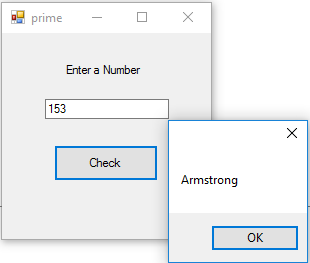# Check whether the Given Number is Armstrong/Strange or Not in VB

Program Abstraction:      This is a VB.Net program which check whether the user typed number is Armstrong number or not with function.

Armstrong Numbers is also known as Strange Numbers. Because these kind of numbers are very strange in number system. So programming Armstrong numbers are really funny and interesting.

#### What is Armstrong Numbers?

These are the numbers, if we take sum of its individual digits with power of number of digits, then result will be same number.
Example:

153
Here number of digit is 3
Then sum of digit with power of number of digit is
1^3 + 5^3 + 3^3 => 1 + 125 + 27 = 153 (Again original number)

8208
Here number of digit is 4
So
8^4 + 2^4 + 0^4 + 8^4 => 4096 + 16 + 0 + 4096 = 8208

All one digit numbers are Armstrong numbers. That is 1,2,3,4…. 9
2^1 = 2
3^1 = 3
9474
5 54748,
6 548834
7 1741725, 4210818, 9800817, 9926315
8 24678050, 24678051, 88593477

Other examples are:

370, 371, 1634, 9474, 54748, 92727, 93084, 548834, 1741725, 4210818, 9800817, 9926315, 24678050, 24678051, 88593477 etc … but very large, rare and strange

Let us see how to write a Visual studio.Net program to check whether the given number is Armstrong or not.

`Private Sub Button1_Click(ByVal sender As System.Object, ByVal e As System.EventArgs) Handles Button1.Click`

Dim num As Integer = 0

Dim rmd As Integer = 0

Dim sum As Integer = 0

Dim orginal As Integer

Dim tempNum As Integer

Dim count As Integer = 0

num = TextBox1.Text

orginal = num

tempNum = num

While tempNum <> 0

count = count + 1

tempNum = Math.Floor(tempNum / 10)

End While

While num > 0

rmd = Math.Floor(num Mod 10)

num = Math.Floor(num / 10)

sum = sum + Math.Pow(rmd, count)

End While
If sum = orginal Then

MessageBox.Show(“Armstrong”)

Else

MessageBox.Show(“Not Armstrong”)

End If

End Sub

At first a loop counts the number of digits and power the individual digits with number of digit and sum it. Finally check the given number with original number. If both are equal then that number should be an Armstrong number.
Math.Floor used to avoid rounding numbers. If we don’t use this function, then 4.2 consider as 4 and 4.6 consider as 5.Program using Function:

`Public Class windowsfn`

Dim original As Integer

Dim sum As Integer = 0

Dim rmd As Integer = 0

Private Sub Button2_Click(ByVal sender As System.Object, ByVal e As System.EventArgs) Handles Button2.Click

Dim number As Integer

Dim rmd As Integer = 0

Dim tempNum As Integer

Dim count As Integer = 0

number = TextBox1.Text

orginal = number

tempNum = number
While tempNum <> 0

count = count + 1

tempNum = Math.Floor(tempNum / 10)

End While

amscheck(number, count)

End Sub

Private Sub amscheck(ByVal num, ByVal count)

sum = 0

While num > 0

rmd = Math.Floor(num Mod 10)

num = Math.Floor(num / 10)

sum = sum + Math.Pow(rmd, count)

End While

If sum = original  Then

MessageBox.Show(“Armstrong Number”)

Else

MessageBox.Show(“Not an Armstrong Number”)

End If

End Sub

End Class

Note that, here we use integer as number data types. So we can’t check large digit number using this program.